# Stability of an elastic system

Jump to: navigation, search

Stability of an elastic system is the property of the elastic system (an elastic body or a system of interacting elastic bodies) of deviating little from an equilibrium position (motion) for sufficiently small disturbing factors. The role of the disturbing factors is played by fluctuations in the external force, deviations from the ideal geometrical shape, material defects, etc.

Stability of an elastic system refers to that part of the mechanics of deformable solids which includes the study of the stability of all deformable systems, like elastic, visco-elastic and elastic-plastic systems; often the term stability of deformable systems is used.

The concept of stability of elastic systems is closely connected with the concept of stability of motion, in particular the concept of Lyapunov stability. The central question in the theory of stability of elastic systems is to find a region in the parameter space of the system with its external actions, within the boundary of which the equilibrium position (motion) can be considered as stable. The surface bounding the region of stability is called the critical surface. Often the action on an elastic system is given up to a parameter. Without loss of generality one may suppose that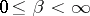, where for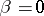there is stability. The lower bound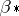of the values of the parameterfor which the equilibrium (motion) considered is stable is called the critical parameter. Problems of the stability of elastic systems have great value in applications: loss of stability of elements of a structure, a machine or an apparatus implies, as a rule, loss of carrying capacity or violation of the normal conditions of service.

The rigorous theory of stability of elastic systems is based on an extension of classical stability theory to continuous systems and may be regarded as an application of the theory of differential equations in Banach spaces. The proximity of the position being studied and the perturbed position is estimated in a certain norm. In practical calculations, one widely uses the approximation of continuous systems by systems with finitely many degrees of freedom, with the wide attraction of variational, grid and other approximate methods.

In the case of an elastic system with ideal constraints, under potential (conservative) time-independent forces, the system in the large is conservative. A condition for the stability of an equilibrium is given by the Lagrange–Dirichlet theorem, according to which in a stable equilibrium position the potential energy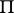of the system has an isolated minimum. On this theorem the energy method for studying the stability of elastic systems is based. It consists in a study of the changes of the potential energyof the system for changes of the parameters. For this,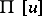is a functional on the displacement field. In a one-parameter problem on the stability of an elastic system the critical parameteris the lower bound of the values offor which the inequality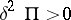, under the condition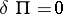, is violated. In a neighbourhood of the critical valuethere is bifurcation of the forms of equilibrium.

For a calculation of the critical parameters corresponding to bifurcation points, instead of the energy method it is usual to employ the static method. Here the problem of the stability of elastic systems is reduced to the linear problem of the eigenvalues for the operator equation corresponding to the variational condition. Here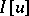is the quadratic functional of the displacement field, which formally coincides withifundergoes the variation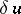. The minimal eigenvalue is taken as critical parameter. As a rule an additional analysis confirms that for an admissible minimal eigenvalue a bifurcation of the forms of equilibrium takes place.

Both methods were first treated in the works of L. Euler on the basis of the classical calculus of variations (1744–1757). He also solved the simpler problem of the stability of prismatic elastic beams under axial pressure, and also studied the behaviour of beams under stability loss. For a freely supported beam, the critical force is equal to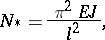whereis the Young modulus of the material,is the moment of inertia of a cross-section andis the length of the beam. There is a large number of concrete results for beams, beam-like systems, plates, shells, as well as for bodies all characteristic dimensions of which having the same order of magnitude (cf. ).

In the case of non-potential forces the energy and static methods are, generally speaking, inapplicable (cf. ). They are also inapplicable for dynamical problems on the stability of elastic systems (cf. ). In all these cases one makes use of the dynamic method, which consists of considering small motions of the system in a neighbourhood of the equilibrium (motion) being investigated. For non-potential forces constant in time the study of stability reduces to the generalized eigenvalue problem relative to the parameters of the system, as well as to the characteristic exponents or complex eigenfrequencies. The dynamic method is based on extending the stability theorem in the first approximation to continuous systems. If for the statement of a concrete problem there are no simplifying assumptions, then as a rule this method gives correct results. In the opposite case there may appear a phenomenon which is known under the name of the paradox of stabilization and destabilization (cf. ). Among the non-conservative problems of the theory of stability of elastic systems a considerable place is taken by problems on aero-elasticity and hydro-elasticity (cf. , , ), as well as by problems on the stability under periodic loadings (cf. ). The latter are closely connected with the theory of parametric resonance for continuous systems.

The generalization of the theory of stability of elastic systems to elasto-plastic systems is related to overcoming the serious difficulties in studying the stability of essentially non-linear non-holonomous continuous systems (cf. ). For systems of materials subject to creep and other hereditary phenomena, it is necessary to make a study of the stability on a finite time interval .